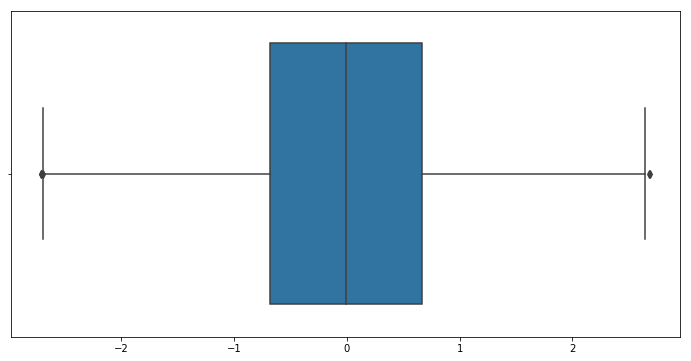# Outlier Detection Using Boxplots

Last updated: January 13th, 2020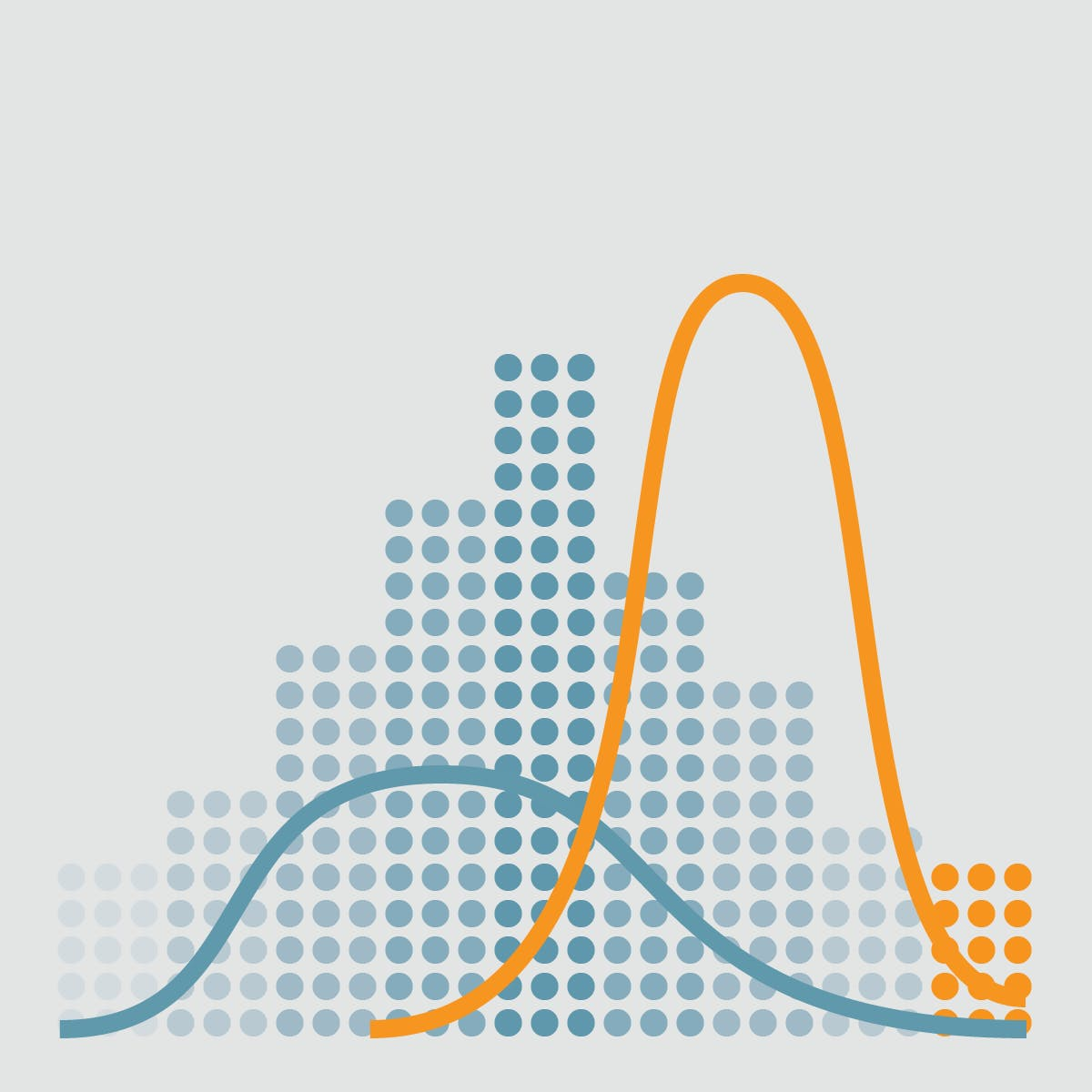# Outlier detection with Boxplots¶

In descriptive statistics, a box plot or boxplot is a method for graphically depicting groups of numerical data through their quartiles. Box plots may also have lines extending vertically from the boxes (whiskers) indicating variability outside the upper and lower quartiles, hence the terms box-and-whisker plot and box-and-whisker diagram.## Hands on!¶

In :
import numpy as np
import pandas as pd
import matplotlib.pyplot as plt
import seaborn as sns

%matplotlib inline## Boxplot¶

A box and whisker plot —also called a box plot— displays five-number summary of a set of data.

Boxplots are a standardized way of displaying the distribution of data based on a five number summary ("minimum", first quartile (Q1), median, third quartile (Q3), and "maximum").

This type of plot is used to easily detect outliers. It can also tell us if your data is symmetrical, how tightly your data is grouped, and if and how your data is skewed.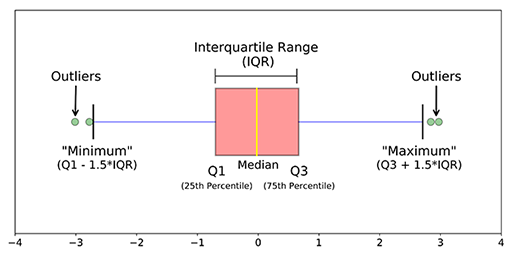• median (Q2/50th Percentile): the middle value of the dataset.
• first quartile (Q1/25th Percentile): the middle number between the smallest number (not the "minimum") and the median of the dataset.
• third quartile (Q3/75th Percentile): the middle value between the median and the highest value (not the "maximum") of the dataset.
• InterQuartile Range (IQR): 25th to the 75th percentile. IQR tells how spread the middle values are.
• "maximum": Q3 + 1.5*IQR
• "minimum": Q1 -1.5*IQR
• Outliers: (shown as green circles) In statistics, an outlier is an observation point that is distant from other observations.

Not every outlier is a wrong value!## Boxplot of a Normal distribution¶

In :
normal = np.random.normal(0, 1, 10000) # loc, scale, size
quartiles = pd.DataFrame(normal).quantile([0.25, 0.5, 0.75, 1])

fig, axs = plt.subplots(nrows=2)
fig.set_size_inches(14, 8)

# Boxplot of Normal distribution
plot1 = sns.boxplot(normal, ax=axs)
plot1.set(xlim=(-4, 4))

# Normal distribution
plot2 = sns.distplot(normal, ax=axs)
plot2.set(xlim=(-4, 4))

# Median line
plt.axvline(np.median(normal), color='r', linestyle='dashed', linewidth=2)

for i, q in enumerate(quartiles):
# Quartile i line
plt.axvline(q, color='g', linestyle='dotted', linewidth=2)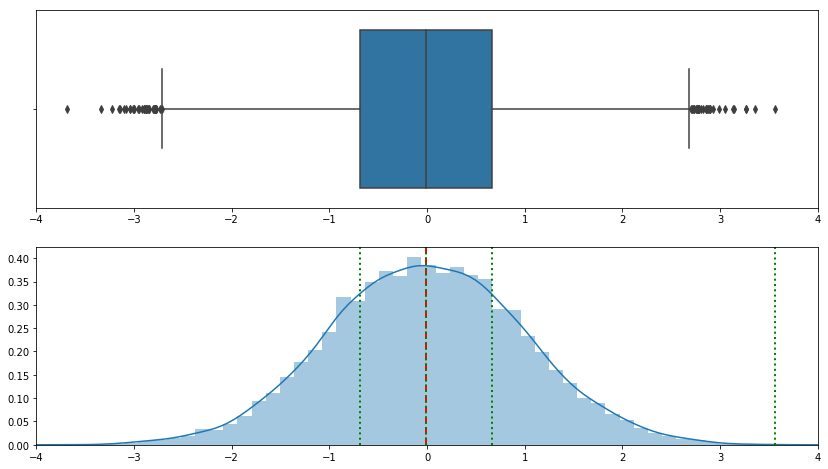## Outliers: drop them or not¶

Most parametric statistics, like means, standard deviations, and correlations, and every statistic based on these, are highly sensitive to outliers. Despite this, it is not acceptable to drop an observation just because it is an outlier. They can be legitimate observations and it's important to investigate the nature of the outlier before deciding whether to drop it or not.

1. DROP: If it is obvious that the outlier is due to incorrectly entered or measured data, you should drop the outlier.

For example a year field with a '9999' value.

1. DROP: If the outlier does not change the results but does affect assumptions, you may drop the outlier. But note that in a footnote of your paper.

Neither the presence nor absence of the outlier in the graph below would change the regression line: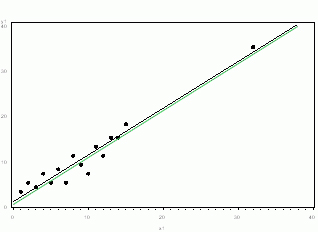1. DROP AND EXPLAIN WHY: More commonly, the outlier affects both results and assumptions. In this situation, it is not legitimate to simply drop the outlier. You may run the analysis both with and without it, but you should state in at least a footnote the dropping of any such data points and how the results changed.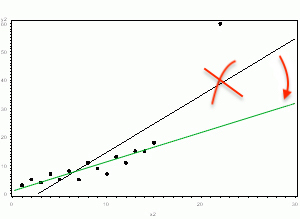1. DROP: If the outlier creates a significant association, you should drop it and should not report any significance from your analysis.

In the following graph, the relationship between X and Y is clearly created by the outlier. Without it, there is no relationship between X and Y, so the regression coefficient does not truly describe the effect of X on Y.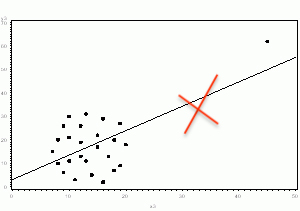### What to do when we shouldn't drop the outlier?¶

• Transformation: you can apply square root or log transformations, that will pull in high numbers.

This can make assumptions work better if the outlier is a dependent variable and can reduce the impact of a single point if the outlier is an independent variable.

• Try a different model: This should be done with caution, but it may be that a non-linear model fits better.

For example, in the above example 3, perhaps an exponential curve fits the data with the outlier intact.

Whichever approach you take, you need to know your data and your research area well. Try different approaches, and see which make theoretical sense.## Removing outliers¶

Now that we know how to build a boxplot and visualize outliers (points outside whiskers), lets remove them:

In :
plt.figure(figsize=(12,6))

sns.boxplot(normal)

Out:
<matplotlib.axes._subplots.AxesSubplot at 0x7f8820c35eb8>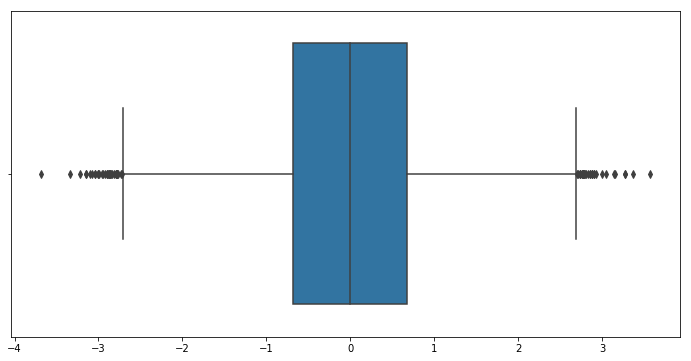Boxplot show us many outliers, but are they wrong values?

We can manually remove values below/above a certain value:

In :
normal[(normal >= -3) & (normal <= 3)]

Out:
array([-0.13228601, -0.43618127,  0.49768295, ..., -0.92085958,
1.1504461 , -0.15994229])
In :
plt.figure(figsize=(12,6))

sns.boxplot(normal[(normal >= -3) & (normal <= 3)])

Out:
<matplotlib.axes._subplots.AxesSubplot at 0x7f8820bacba8>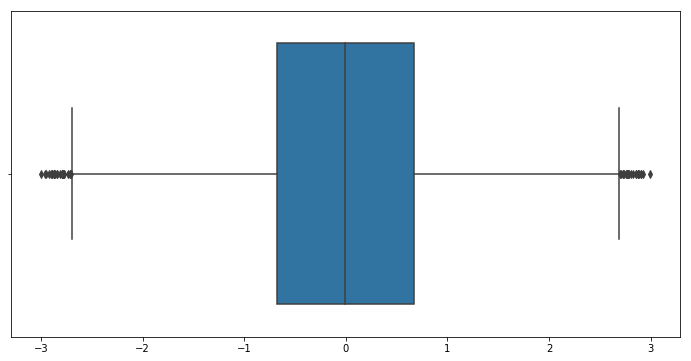Or use low and high fences of the boxplot and remove outer elements:

In :
q1 = pd.DataFrame(normal).quantile(0.25)
q3 = pd.DataFrame(normal).quantile(0.75)
iqr = q3 - q1 #Interquartile range

fence_low = q1 - (1.5*iqr)
fence_high = q3 + (1.5*iqr)

In :
iqr

Out:
1.3531456117664944
In :
fence_low

Out:
-2.7145351881861703
In :
fence_high

Out:
2.698047258879807
In :
# "Outside" boxplot Reviews
normal[(normal < fence_low) | (normal > fence_high)].shape

Out:
66

Keep just the "inside" boxplot points:

In :
plt.figure(figsize=(12,6))

sns.boxplot(normal[(normal >= fence_low) & (normal <= fence_high)])

Out:
<matplotlib.axes._subplots.AxesSubplot at 0x7f8820b40dd8>